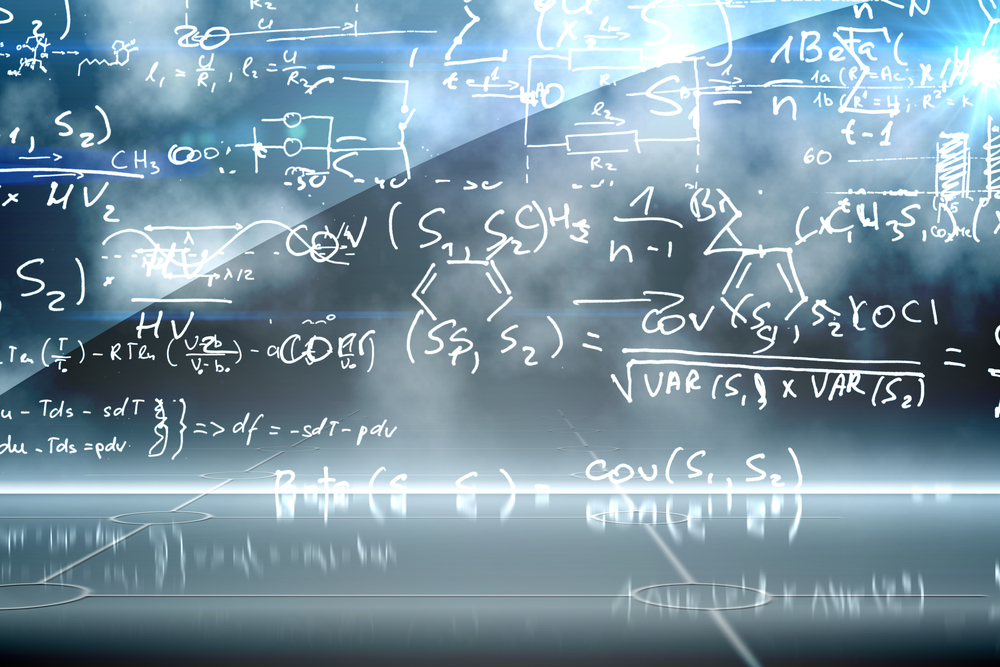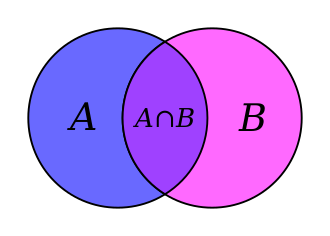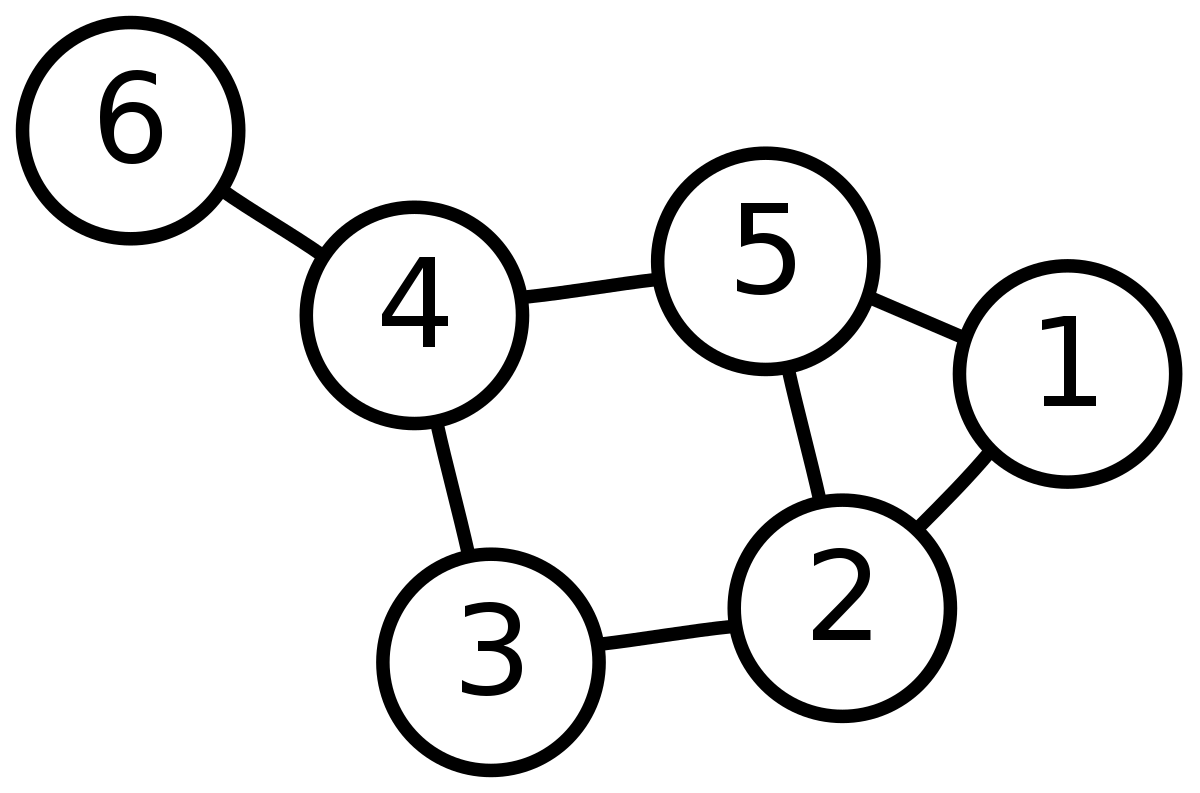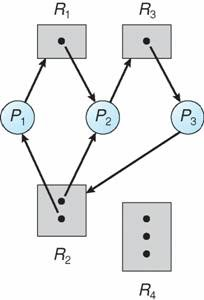# Crazy Math in Cyber Security

“We all use math every day;

to predict weather, to tell time, to handle money.

Math is more than formulas or equations;

it’s logic, it’s rationality,

it’s using your mind to solve the biggest mysteries we know.”

This quote is from a famous crime television show called Numb3rs, where a set of brothers rely on Math to solve crimes in their neighborhood. The math used ranges from statistical probabilities to mapping out timelines. Similar to crime, Math is found just about anywhere, including cybersecurity.## Math is Everywhere

It’s impossible to go a day without interacting with Math in some way, (yes our teachers did have a point). Math is in music, flowers, medicine, weather, time, money, etc. The foundations of computing are in Math and computers, as we know them today wouldn’t exist without Math.

While the everyday cybersecurity specialist probably doesn’t sit at their desk doing calculus, they do use a computer. Many of the principles of cybersecurity are based around encryption, cryptography, and cryptanalysis. Artificial Intelligence and Machine Learning require an extensive understanding of mathematical fields like statistics, linear algebra, multivariable calculus, and probability theory.  Let’s look at some cybersecurity Math examples.

## Discrete Mathematics

Discrete Mathematics is a field of math that focuses on “mathematical structures that are countable or otherwise distinct and separable” (brilliant.org). This field of math is the foundation of computer science. Discrete math has yielded many byproducts, including logical symbols and notation, set theory, and graph theory.

Logical symbols and notation are a way to perform operations on logical values. Some of the most common and recognizable include ∧ for “and”, ∨ for “or”, and ¬ for “not”. So, the expression A∧¬B reads “A and not B”. If A was equal to “true” and B was “equal” to false, the expression would read “true and not false,” the ‘not false’ can be translated as the opposite of false so, now the expression reads “true and true”, which evaluates to true. Recognize some of these examples? These concepts are some of the fundamentals behind conditional and boolean logic and coding. Without which computer science, this would be much more difficult, if not impossible. More symbols and their meanings can be found here.

## Set Theory

Set theory is the study of sets or collections of objects. They are typically objects that are relevant to math, but the theories would apply to almost any grouping of objects. One classic example of set theory is Venn Diagrams. There are operators for this kind of math too! They include ∪ or “union”, ∩ or “intersection”, ⊆ which denotes a subset, and  which is a special kind of difference or subtraction. A figure with a Venn Diagram showing how the union works is in the picture below. Data structures can be thought of as sets, and these operations can apply to them. A database is many sets of data in one location that have particular functions that can be used to search, find, and obtain additional data. Cybersecurity analysts are no stranger to databases, they live in them!## Graph Theory

Graph theory is one of my personal favorites. Graph theory is the study of graphs, but they are not the graphs as you would typically think of them. Mathematical graphs consist of nodes and edges. They represent how things are connected.There are many applications of this in computer science and cybersecurity. One example is in the operating system. Graphs represent how different processes in a system allocate resources, as known as a resource allocation graph.These graphs can determine if there is a deadlock in the system; this when a system is in a state where nothing can get done. There are many reasons this may happen, including systems misfiring or even cyberattacks. Deadlocks can be particularly dangerous for specific systems that are required to be functioning continuously.

*Looking to read a specific example of how graphs are used in data structures? Read my Introduction to Binary Search Trees here.

## Cryptography

We can’t have a blog on Math in Cybersecurity without talking about cryptography! Cryptography is a method of protecting data. The processes behind it are beyond the scope of this article, but most modern cryptography algorithms rely heavily on prime number factorizations. The faster someone can factor prime numbers, the faster they can break the encryption. Mathematicians are working to defend against cyberattacks similar to this, as computer speed and quantum computing are a real threat.

## Summary

Computer science and cybersecurity wouldn’t exist without Math. While not every computer programmer or cybersecurity professional sits at a desk, punching numbers, and writing proofs, math is still vital to their profession. All in all, Math is a way of processing data, which we can all agree, is a cybersecurity analyst’s primary goal.

Resources:

https://brilliant.org/wiki/discrete-mathematics/

https://www.geeksforgeeks.org/what-is-the-importance-of-mathematics-in-computer-science/